Related Articles

# Window to Viewport Transformation in Computer Graphics with Implementation

• Difficulty Level : Medium
• Last Updated : 04 Jun, 2021

Window to Viewport Transformation is the process of transforming 2D world-coordinate objects to device coordinates. Objects inside the world or clipping window are mapped to the viewport which is the area on the screen where world coordinates are mapped to be displayed.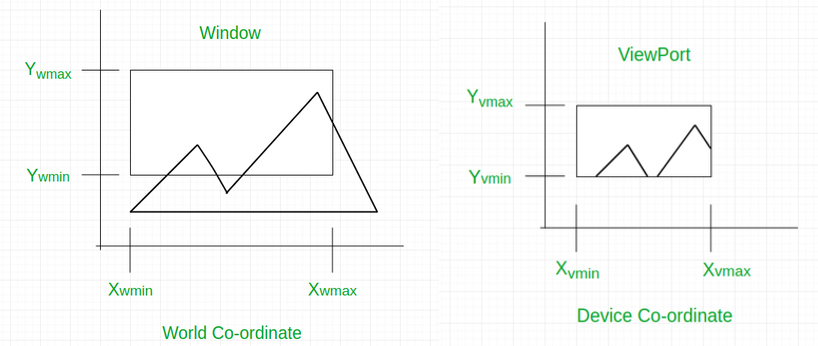General Terms

• World coordinate – It is the Cartesian coordinate w.r.t which we define the diagram, like Xwmin, Xwmax, Ywmin, Ywmax
• Device Coordinate –It is the screen coordinate where the objects are to be displayed, like Xvmin, Xvmax, Yvmin, Yvmax
• Window –It is the area on world coordinate selected for display.
• ViewPort –It is the area on the device coordinate where graphics is to be displayed.

Mathematical Calculation of Window to Viewport:
It may be possible that the size of the Viewport is much smaller or greater than the Window. In these cases, we have to increase or decrease the size of the Window according to the Viewport and for this, we need some mathematical calculations.

```(xw, yw): A point on Window
(xv, yv): Corresponding  point on Viewport```
• we have to calculate the point (xv, yv)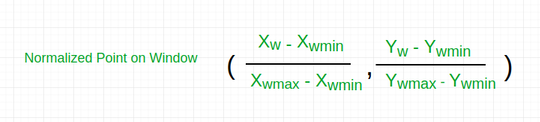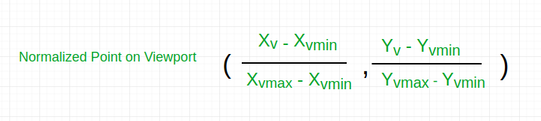• Now the relative position of the object in Window and Viewport are same.
`For x coordinate,                        `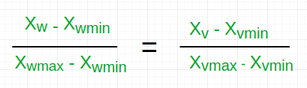` For y coordinate,`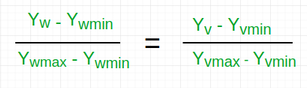• so, after calculating for x and y coordinate, we get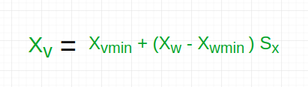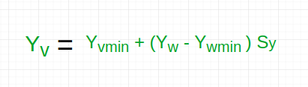• where sx is the scaling factor of x coordinate and sy is the scaling factor of y coordinate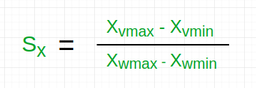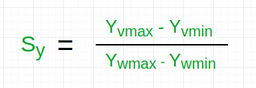Example:
Lets assume,

• for window, Xwmin = 20, Xwmax = 80, Ywmin = 40, Ywmax = 80.
• for viewport, Xvmin = 30, Xvmax = 60, Yvmin = 40, Yvmax = 60.
• Now a point ( Xw, Yw ) be ( 30, 80 ) on the window. We have to calculate that point on the viewport
i.e ( Xv, Yv ).
• First of all, calculate the scaling factor of x coordinate Sx and the scaling factor of y coordinate Sy using the above-mentioned formula.
```Sx = ( 60 - 30 ) / ( 80 - 20 ) = 30 / 60
Sy = ( 60 - 40 ) / ( 80 - 40 ) = 20 / 40```
• So, now calculate the point on the viewport ( Xv, Yv ).
```Xv = 30 + ( 30 - 20 ) * ( 30 / 60 ) = 35
Yv = 40 + ( 80 - 40 ) * ( 20 / 40 ) = 60```
• So, the point on window ( Xw, Yw ) = ( 30, 80 ) will be ( Xv, Yv ) = ( 35, 60 ) on viewport.

Here is the implementation of the above approach:

## C++

 `// C++ program to implement``// Window to ViewPort Transformation` `#include ``using` `namespace` `std;` `// Function for window to viewport transformation``void` `WindowtoViewport(``int` `x_w, ``int` `y_w, ``int` `x_wmax,``                      ``int` `y_wmax, ``int` `x_wmin, ``int` `y_wmin,``                      ``int` `x_vmax, ``int` `y_vmax, ``int` `x_vmin,``                      ``int` `y_vmin)``{``    ``// point on viewport``    ``int` `x_v, y_v;` `    ``// scaling factors for x coordinate and y coordinate``    ``float` `sx, sy;` `    ``// calculating Sx and Sy``    ``sx = (``float``)(x_vmax - x_vmin) / (x_wmax - x_wmin);``    ``sy = (``float``)(y_vmax - y_vmin) / (y_wmax - y_wmin);` `    ``// calculating the point on viewport``    ``x_v = x_vmin + (``float``)((x_w - x_wmin) * sx);``    ``y_v = y_vmin + (``float``)((y_w - y_wmin) * sy);` `    ``cout<< ``"The point on viewport: ("``<

## C

 `// C program to implement``// Window to ViewPort Transformation` `#include ` `// Function for window to viewport transformation``void` `WindowtoViewport(``int` `x_w, ``int` `y_w, ``int` `x_wmax,``                      ``int` `y_wmax, ``int` `x_wmin, ``int` `y_wmin,``                      ``int` `x_vmax, ``int` `y_vmax, ``int` `x_vmin,``                      ``int` `y_vmin)``{``    ``// point on viewport``    ``int` `x_v, y_v;` `    ``// scaling factors for x coordinate and y coordinate``    ``float` `sx, sy;` `    ``// calculating Sx and Sy``    ``sx = (``float``)(x_vmax - x_vmin) / (x_wmax - x_wmin);``    ``sy = (``float``)(y_vmax - y_vmin) / (y_wmax - y_wmin);` `    ``// calculating the point on viewport``    ``x_v = x_vmin + (``float``)((x_w - x_wmin) * sx);``    ``y_v = y_vmin + (``float``)((y_w - y_wmin) * sy);` `    ``printf``(``"The point on viewport: (%d, %d )\n "``, x_v, y_v);``}` `// Driver Code``void` `main()``{``    ``// boundary values for window``    ``int` `x_wmax = 80, y_wmax = 80, x_wmin = 20, y_wmin = 40;` `    ``// boundary values for viewport``    ``int` `x_vmax = 60, y_vmax = 60, x_vmin = 30, y_vmin = 40;` `    ``// point on window``    ``int` `x_w = 30, y_w = 80;` `    ``WindowtoViewport(30, 80, 80, 80, 20, 40, 60, 60, 30, 40);``}` `//this code is added by khushboogoyal499`

## Java

 `// Java program to implement``// Window to ViewPort Transformation``class` `GFG``{` `// Function for window to viewport transformation``static` `void` `WindowtoViewport(``int` `x_w, ``int` `y_w, ``int` `x_wmax,``                    ``int` `y_wmax, ``int` `x_wmin, ``int` `y_wmin,``                    ``int` `x_vmax, ``int` `y_vmax, ``int` `x_vmin,``                    ``int` `y_vmin)``{``    ``// point on viewport``    ``int` `x_v, y_v;` `    ``// scaling factors for x coordinate and y coordinate``    ``float` `sx, sy;` `    ``// calculating Sx and Sy``    ``sx = (``float``)(x_vmax - x_vmin) / (x_wmax - x_wmin);``    ``sy = (``float``)(y_vmax - y_vmin) / (y_wmax - y_wmin);` `    ``// calculating the point on viewport``    ``x_v = (``int``) (x_vmin + (``float``)((x_w - x_wmin) * sx));``    ``y_v = (``int``) (y_vmin + (``float``)((y_w - y_wmin) * sy));` `    ``System.out.printf(``"The point on viewport: (%d, %d )\n "``, x_v, y_v);``}` `// Driver Code``public` `static` `void` `main(String[] args)``{` `    ``// boundary values for window``    ``int` `x_wmax = ``80``, y_wmax = ``80``, x_wmin = ``20``, y_wmin = ``40``;` `    ``// boundary values for viewport``    ``int` `x_vmax = ``60``, y_vmax = ``60``, x_vmin = ``30``, y_vmin = ``40``;` `    ``// point on window``    ``int` `x_w = ``30``, y_w = ``80``;` `    ``WindowtoViewport(``30``, ``80``, ``80``, ``80``, ``20``, ``40``, ``60``, ``60``, ``30``, ``40``);``}``}` `// This code is contributed by Rajput-Ji`

## Python3

 `# Python3 program to implement``# Window to ViewPort Transformation` `# Function for window to viewport transformation``def` `WindowtoViewport(x_w, y_w, x_wmax, y_wmax,``                       ``x_wmin, y_wmin, x_vmax,``                       ``y_vmax, x_vmin, y_vmin):``                           ` `    ``# point on viewport``    ``# calculating Sx and Sy``    ``sx ``=` `(x_vmax ``-` `x_vmin) ``/` `(x_wmax ``-` `x_wmin)``    ``sy ``=` `(y_vmax ``-` `y_vmin) ``/` `(y_wmax ``-` `y_wmin)` `    ``# calculating the point on viewport``    ``x_v ``=` `x_vmin ``+` `((x_w ``-` `x_wmin) ``*` `sx)``    ``y_v ``=` `y_vmin ``+` `((y_w ``-` `y_wmin) ``*` `sy)` `    ``print``(``"The point on viewport:("``, ``int``(x_v),``                                ``","``, ``int``(y_v), ``")"``)` `# Driver Code``if` `__name__ ``=``=` `'__main__'``:``    ` `    ``# boundary values for window``    ``x_wmax ``=` `80``    ``y_wmax ``=` `80``    ``x_wmin ``=` `20``    ``y_wmin ``=` `40` `    ``# boundary values for viewport``    ``x_vmax ``=` `60``    ``y_vmax ``=` `60``    ``x_vmin ``=` `30``    ``y_vmin ``=` `40` `    ``# point on window``    ``x_w ``=` `30``    ``y_w ``=` `80` `    ``WindowtoViewport(``30``, ``80``, ``80``, ``80``, ``20``,``                     ``40``, ``60``, ``60``, ``30``, ``40``)``    ` `# This code is contributed by Surendra_Gangwar`

## C#

 `// C# program to implement``// Window to ViewPort Transformation``using` `System;` `class` `GFG``{` `// Function for window to viewport transformation``static` `void` `WindowtoViewport(``int` `x_w, ``int` `y_w,``                             ``int` `x_wmax, ``int` `y_wmax,``                             ``int` `x_wmin, ``int` `y_wmin,``                             ``int` `x_vmax, ``int` `y_vmax,``                             ``int` `x_vmin, ``int` `y_vmin)``{``    ``// point on viewport``    ``int` `x_v, y_v;` `    ``// scaling factors for x coordinate``    ``// and y coordinate``    ``float` `sx, sy;` `    ``// calculating Sx and Sy``    ``sx = (``float``)(x_vmax - x_vmin) /``                ``(x_wmax - x_wmin);``    ``sy = (``float``)(y_vmax - y_vmin) /``                ``(y_wmax - y_wmin);` `    ``// calculating the point on viewport``    ``x_v = (``int``) (x_vmin +``        ``(``float``)((x_w - x_wmin) * sx));``    ``y_v = (``int``) (y_vmin +``        ``(``float``)((y_w - y_wmin) * sy));` `    ``Console.Write(``"The point on viewport: "` `+``                 ``"({0}, {1} )\n "``, x_v, y_v);``}` `// Driver Code``public` `static` `void` `Main(String[] args)``{` `    ``// boundary values for window``    ``int` `x_wmax = 80, y_wmax = 80,``        ``x_wmin = 20, y_wmin = 40;` `    ``// boundary values for viewport``    ``int` `x_vmax = 60, y_vmax = 60,``        ``x_vmin = 30, y_vmin = 40;` `    ``// point on window``    ``int` `x_w = 30, y_w = 80;` `    ``WindowtoViewport(30, 80, 80, 80, 20,``                     ``40, 60, 60, 30, 40);``}``}` `// This code is contributed by PrinciRaj1992`

## Javascript

 ``
Output:
`The point on viewport: (35, 60 )`

Attention reader! Don’t stop learning now. Get hold of all the important DSA concepts with the DSA Self Paced Course at a student-friendly price and become industry ready.  To complete your preparation from learning a language to DS Algo and many more,  please refer Complete Interview Preparation Course.

In case you wish to attend live classes with experts, please refer DSA Live Classes for Working Professionals and Competitive Programming Live for Students.

My Personal Notes arrow_drop_up